Facilitating the spread of knowledge and innovation in professional software development

Contribute

### Topics

InfoQ Homepage Articles Approximate Queries on WSO2 Stream Processor: Use of Approximation Algorithms in an Applied Setting

# Approximate Queries on WSO2 Stream Processor: Use of Approximation Algorithms in an Applied Setting

### Key Takeaways

• Large amounts of big data produced in data streams makes it impossible to compute exact answer for stream processing queries.
• Approximate computing has gained significant attention in recent times due to its ability of producing “fit-for-the-purpose” results with lower resource footprints.
• Data stream processing applications can produce estimates of the results by using approximate computing algorithms/data structures such as HyperLogLog, Count-min Sketch, etc.
• It is essential to select appropriate approximate computing technique to operate with stream processing windows in-order to ensure reliable operation of the system.
• Benefits of the use of approximate computing becomes visible mostly with high data rate streams.

## 1. Introduction

Data stream processing has become one of the main paradigms for data analytics in recent times due to the growing requirement of stream processing applications. Different recent applications of stream processing are available in areas such as telecommunications, vehicle traffic management, crowd management, health informatics, Cyber security, finance, etc.

The vast amounts of streaming data introduce the challenge of handling the data deluge. Multiple solutions exist for this problem, such as upgrading the system hardware, additional resource provisioning via elastic scaling into external clouds, discarding events randomly, approximate computing, etc. Out of these alternatives approximate computing becomes a promising approach compared to the others since it does not require additional resource provisioning.

Approximate computing becomes important due to several reasons. First, approximate output is good enough for many use cases. Especially, when the trend data is more important than the precise numbers. An example of this is knowing the number of customers visited a website like “eBay”, which has billions of customers. Often you don’t need to know the number of customers that visited your site in the last hour exactly. It may take few minutes to compute the exact number. Instead knowing an approximate answer within a second will be more useful.

Second, The relaxing of the accuracy requirements enables one to save multiple resources such as CPU cycles and memory. Such savings of system resources are vital to implement practical IoT (Internet of things) applications where the devices at the edge operate with low power envelopes. Furthermore, it enables discovering large datasets.

In this article, we describe an example real world application of API monitoring which gets benefit by using approximate stream processing. We developed the application on top of WSO2 Stream Processor as Siddhi extension. Siddhi is the complex event processing library which acts as the event processing engine of WSO2 Stream Processor.

The HTTP requests to a specific API from different clients are passed to the stream processor to analyze the number of unique users (See Figure 1). The results are used to balance the load of API and the number accesses of each client which can be used to categorize the clients. We conducted the test using a stream of randomly generated IP addresses. We defined the input stream as follows,

define stream requestStream(ip String, timestamp long);

Listing 1: Definition of the input stream.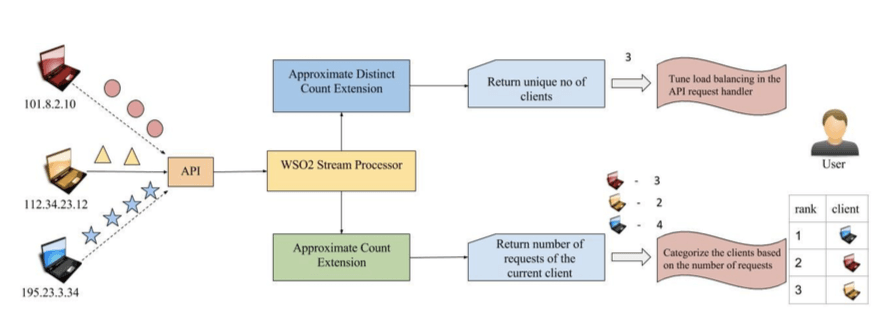Figure 1: Monitoring the API access statistics.

We observed improvements of throughput values of 144% and 66%, and improvements in latency values of 78% and 45% respectively for Distinct Count and Count functions. The results were produced within +/- 5% error margin which means the results are produced within 5% accuracy. Here, the upper and the lower bounds for the results of this process are calculated as follows,

lower bound result = approx result - (approx result * 0.05)


Listing 2: How the lower and upper bounds for the results have been defined.

The error margin has been determined by two configuration parameters called relative error and confidence. In order to achieve an error margin of +/- 5% a relative error of 0.1 and confidence of 0.95 was utilized. We choose +/- 5% as the error margin because we can use Java Integers (which are 32 bit) to represent hashes. The 5% error corresponds to 400+ buckets where the bucket id length is 9 bits. This is close to 1/4 of 32 which is the size of the integer hash we are using. The approximate computing functionalities described in this article have been released as open source software.

In this article we first describe the techniques we adapt for implementing the approximate computing (i.e., HyperLogLog and Count-min Sketch). Next, we describe the implementation of our approach and the experiments conducted to prove the benefits of approximate computing.  We found that the results are quite promising where approximate computing can deliver results with consistent higher throughput and lower latencies compared to the naive approaches. The article concludes with Summary section highlighting these benefits.

## 2. Techniques for Approximate Computing

Currently two approximate computing algorithms have been used for implementing the approximate computing functionality in the WSO2 Stream Processor. These are HyperLogLog and Count min-sketch algorithms which are described in the next subsections respectively.

### 2.1 Approximate Distinct Count: HyperLogLog

HyperLogLog is an algorithm which is used to count the approximate cardinality (i.e., number of distinct elements) of items in a set. In contrast to other approaches, it uses a data sketch to keep track of counts instead of keeping all the unique items within the set. The main concept of the algorithm is counting the unique items using recurring probability of specific bit patterns in a set of bit strings. Therefore, before updating the sketch the items are converted to bit strings using hash functions. Multiple recent applications of HyperLogLog have appeared in recent times such as detection of worm propagation, detection of network attacks, network traffic monitoring, etc.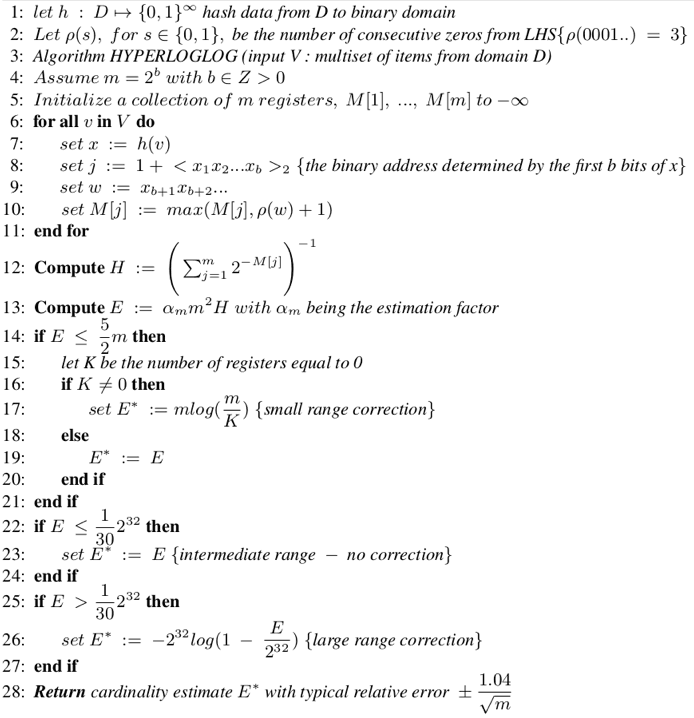Algorithm 1: The HyperLogLog Algorithm

HyperLogLog algorithm has been shown above (See Algorithm 1). The input to the algorithm is a multiset V of data items. HyperLogLog’s output is an estimate of the cardinality which is the number of distinct elements in V. HyperLogLog algorithm uses an integer array of fixed size(b) used to keep the count of cardinality(distinct count of items) of a set.

HyperLogLog uses the concept of occurrence of patterns. Let’s consider the scenario of b=4 where we consider the first four bits from the left hand side (LHS) of the bit string.   If a bit string of 0000xxx… is seen, this string has a probability of 1/16 (=1/(2⁴)) of occurring.The 0000 pattern had a good chance of being seen at any point within 6.25%(100 x 1/16) of the set. There is a higher chance  16 (=2⁴) unique strings should have been occurred. Therefore, by keeping the largest bit pattern of the inserted values, an approximation for the number of unique values can be obtained.

A single estimate in HyperLogLog can be skewed. Therefore, to improve the approximation, inputs are categorized into different buckets(array cells) by using a fixed size prefix of them and the final estimate is calculated using an averaging technique. In the below example, the prefix is 3 bits in length. Then the remaining part of the input is used to find the largest bit pattern(e.g., consecutive number of zeros).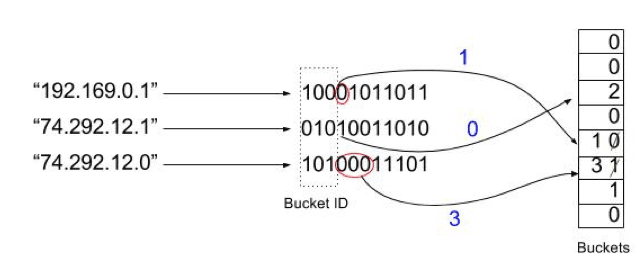Figure 2: An example of the HyperLogLog functionality

Insertion of values happens as follows. Consider the three IP address values shown in Figure 2. First, they are converted to bit strings via a hash function. Here, the first 3 bits are considered as bucket ids. Then the number of consecutive zeros in the remaining part of bit string is entered into the buckets unless the values already in the buckets are bigger than them.

Querying values happens by calculating the harmonic mean of the values in all the buckets.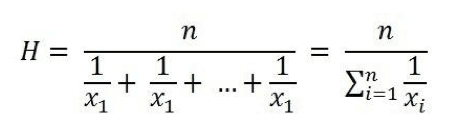Listing 1: Formula for Harmonic Mean

Harmonic mean formula is shown in Listing 1. Harmonic mean is used to normalize the result. Where,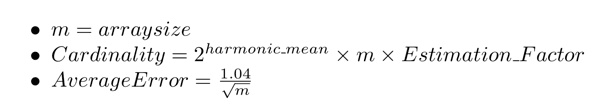### 2.2 Approximate count: Count-min Sketch

Count-min Sketch algorithm uses a 2D integer array to keep count of the values entered to a certain set. The width of the array is w and its height d  represents the number of hash functions used (See Figure 3).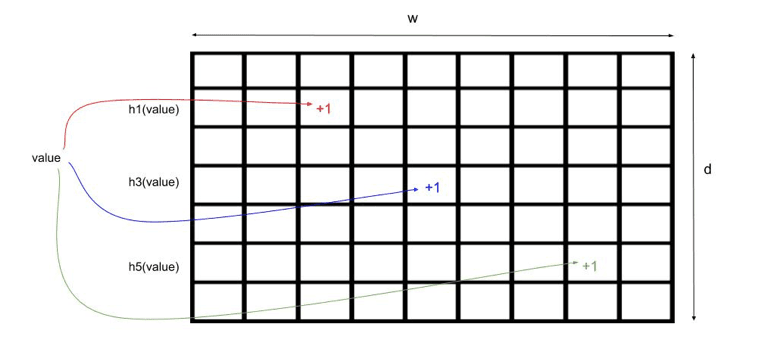Figure 3: The 2D integer array used by Count-min Sketch algorithm

The parameters w and d are selected by considering the accuracy of the output which is expected. Specifically the w and d can be calculated as,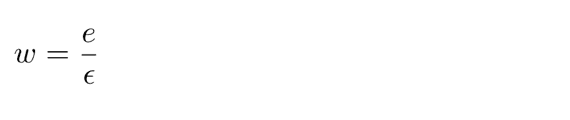Listing 2: Width of the 2D array of Count-min Sketch

Where e is the base of the natural logarithm and ε is the relative error.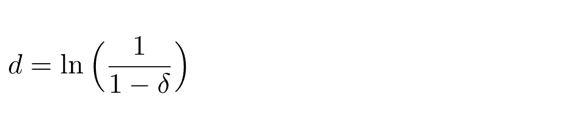Listing 3: Height of the 2D array of Count-min Sketch

Where δ is the confidence with which the answers have the specified relative error.

Inserting a value happens as follows. Consider d number of hash functions (h0, h1,…) where each hash function maps the values to one of values in 0, 1,…, w-1. Consider the hash values obtained for a value is h1(value) = 2, ... ,h3(value) = 4, …,  h5(value) = 7, … . Here each value in the cells relevant for the hash functions is incremented by one  (See Figure 3).

Querying a value from the 2D array happens as follows. Consider if you want to know the count of value x. Then all the hash values of x are calculated as h1(x) = 1, h2(x) =3 , …, hd(x) = 2. The minimum value in the cells of those values are taken as the answer.

Count = min{cell_value(h1(x)), cell_value(h2(x)), …, cell_value(hd(x))}

Listing 4: Height of the 2D array of Count-min Sketch

Count-min Sketch algorithm does not underestimate the value. But overestimating can happen due to the mapping of different values to the same cell by the hash functions.

### 3. Our Approach

In this section we provide the details of how we implemented the HyperLogLog and Count-Min-Sketch algorithm as Siddhi extensions. Both distinctCount and count extensions were implemented as Stream Processor extensions.

The approximate distinctCount extension (shown in Figure 4) processes the incoming events from an input stream and updates a data sketch(HyperLogLog data structure). Then the current distinct count value is calculated using the data stored in the sketch and returned with the output events.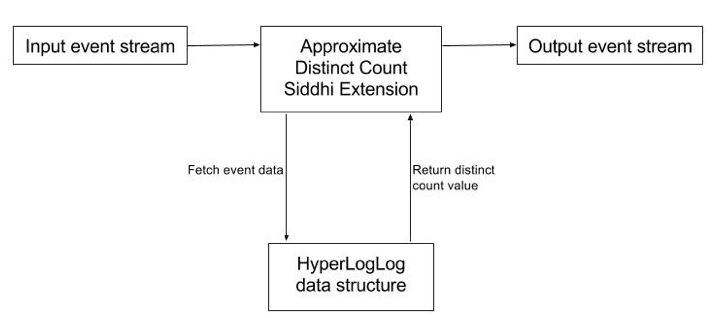Figure 4: Architecture of the Approximate Distinct Count Siddhi extension

The approximate count extension (shown in Figure 5) processes the incoming events from an input stream and update a data sketch(Count-Min-Sketch data structure). Then the current count value relevant to the incoming event is calculated using the data stored in the sketch and returned with the output event.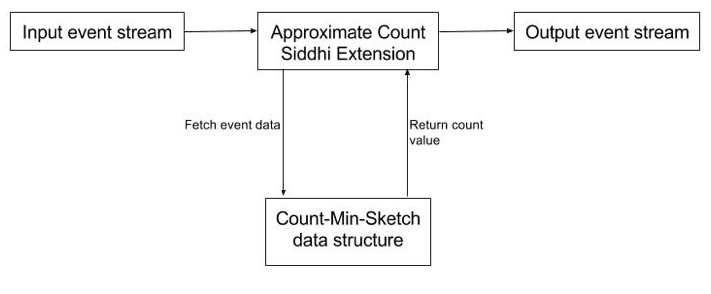Figure 5: Architecture of the Approximate Count Siddhi extension

Unlike the conventional application of approximate computing (such as databases), use of approximate computing techniques such as Distinct Count and Count have to be done carefully in the case of use of event windows. Both the techniques could operate normally by default with event windows. However, Distinct Count may consume excessive amounts of system’s memory if a window has not been used in front of the Distinct Count operation.This is because windows by default causes the events accumulated within the Distinct Count component to expire. However, if a window has not been used such expiration does not get triggered. This causes the events to get infinitely accumulated within the Distinct Count extension.

## 4. Experiments

In the example application described in the Introduction section, we are interested in measuring the capability of our application to handle large number of HTTP requests received per second. In order to simulate the above mentioned scenario we implemented a client application which generates random IP values. We also implemented another application which uses Siddhi stream processor to process events sent by client and calculate the distinct count and count values. Note that the random IP values generated could be repeating in the dataset.

The output throughput and latency values were measured for four different cases.

1. Using non-approximate(exact) Siddhi query to calculate distinct count of events
2. Using approximate Siddhi query to calculate distinct count of events
3. Using non-approximate(exact) Siddhi query to calculate count(frequency) of events
4. Using approximate Siddhi query to calculate count(frequency) of events

The performance results of the case 1 were compared against the case 2 and the results of the case 3 were compared against the case 4.

The application client and the server were ran in two computers which were connected using an Ethernet cable and the events were sent via a TCP channel. The computer(Lenovo T520 laptop) running the application client was configured with Intel  Core i7-2630M, 2.00GHz, 8 cores and 8GB RAM. The computer(Lenovo T530 laptop) running the server was configured with Intel  Core i7-3520M, 2.90GHz, 4 cores and 8GB RAM. Both the computers were installed with Linux Ubuntu(16.04 LTS) 64-bit version, Siddhi 4.0.0, and Oracle JDK 1.8. The maximum and minimum heap of 4GB was allocated for the JVMs of  both computers.

The experiments were categorized for measuring performance of approximate distinct count extension and approximate count extension. For each category, we ran a script to fetch events to a Siddhi event stream. To observe the performance of the naive approach, the event stream was passed through a Siddhi query which uses the naive approaches to calculate the counts. The throughput and latency values were calculated based on the output events of the query.

For measuring the performance of the extension, the Siddhi query was changed to use the approximate extension and followed the same procedure to measure the throughput and latency. Note that the throughput and latency were measured for each 1 million events output from the Siddhi queries. Hence the window size used in these experiments were 1 million. The queries used for the experiments are shown in Table 1.

Table 1: Comparison of the queries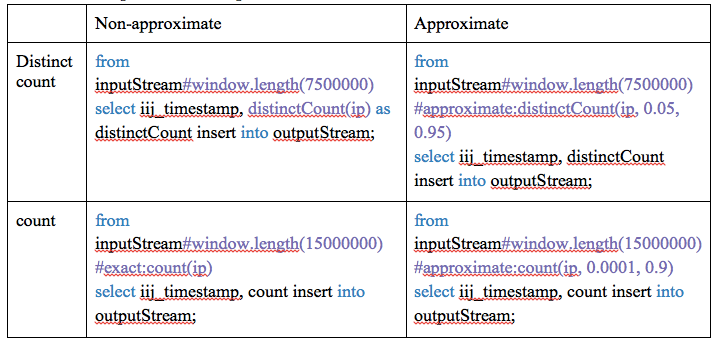## 5. Results

When using the non-approximate approach to calculate the distinct count of events, the reported overall throughput value was about 21,312 events/second and the entire average latency per event was about 0.02424607ms. It should be noted that the input events have been sent at the maximum rate where the non-approximate approach could handle the input data rate. This is because use of such high input data rate could clearly indicate the benefits of use of approximate computing techniques. When using the approximate distinct count extension, the reported overall throughput value was about 52,012 events/second which is a 144% improvement and the entire average latency per event was about 0.00522114ms which is a 78% improvement. The average throughput and average latency variations of the distinct count queries are shown in Figures 6 and 7 respectively. It can be observed that although both throughput and latency curves start at similar values, the non-approximate algorithm’s performance degrades soon while the approximate version maintains the same performance from the beginning till the end of the experiment.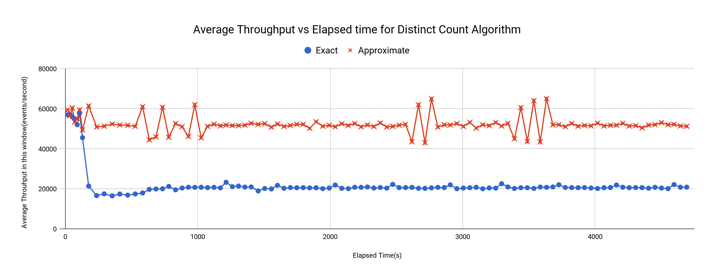Figure 6 : Average throughput variation for distinct count queries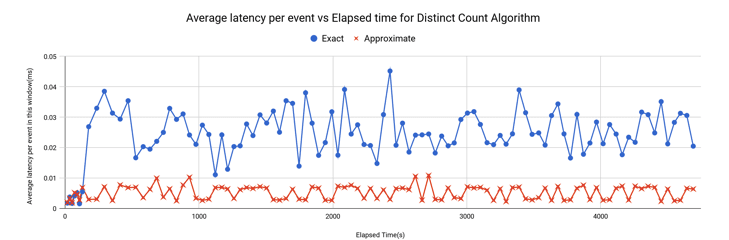Figure 7 : Average latency variation for distinct count queries

When using the non-approximate approach to calculate the count(frequency) of events, the reported overall throughput value was about 32,390 events/second and entire average latency per event was about 0.0087243ms. When using the approximate count extension, the reported overall throughput value was about 54,070 events/second which is a 66% improvement and entire average latency per event was about 0.00475573ms which is a 45% improvement. The average throughput and average latency variation of the count queries are shown in Figures 8 and 9 respectively. A similar behavior to the distinct count performance curves could be observed with the count queries.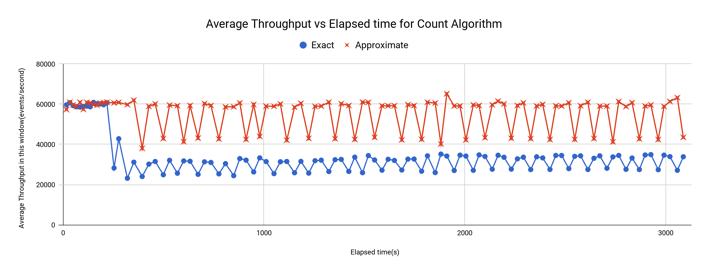Figure 8 : Average throughput variation for count queries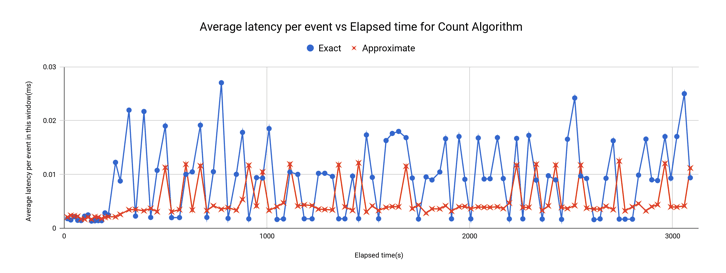Figure 9 : Average latency variation for count queries

The overall results of the above charts could be summarized as shown in Table 2.

Table 2: Summary of the experiment results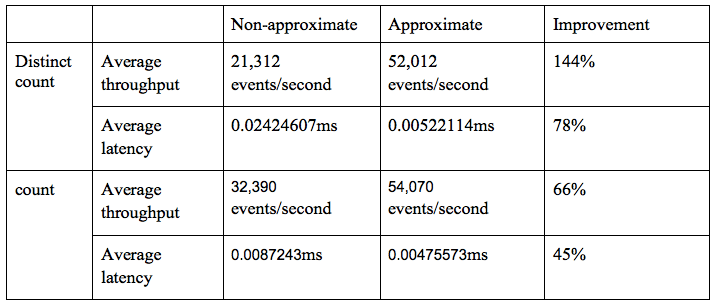## 6. Summary

Increasingly approximate computing is becoming a mainstream technology for data stream processing which provides efficient use of system resources compared to exact processing approaches. In this article we described one example where approximate computing techniques have been applied for an API monitoring application. The results are quite promising where approximate computing can deliver results with consistent higher throughput and lower latencies compared to the naive approaches. We also point out the importance of careful designing of the approximate stream processing extensions to make them operate reliably. Both Distinct count and Count extensions have been developed as WSO2 Siddhi extensions. The extensions are accessible under Apache 2.0 open source license from .

## About the AuthorsChamod Samarajeewa is an Intern Software Engineer at WSO2. He is currently a third year undergraduate at the Department of Computer Science and Engineering, University of Moratuwa, Sri Lanka. His research interests include data stream processing and approximate computing.Miyuru Dayarathna is a Senior Technical Lead at WSO2. He is a computer scientist with multiple research interests and contributions in stream computing, graph data management and mining, cloud computing, performance engineering, IoT, etc. He is also a consultant at the Department of Computer Science and Engineering, University of Moratuwa, Sri Lanka. He has published technical papers in reputed international journals and conferences as well as organized several international workshops on high performance graph data management and processing.

Style

## Hello stranger!

You need to Register an InfoQ account or or login to post comments. But there's so much more behind being registered.

Get the most out of the InfoQ experience.

Allowed html: a,b,br,blockquote,i,li,pre,u,ul,p